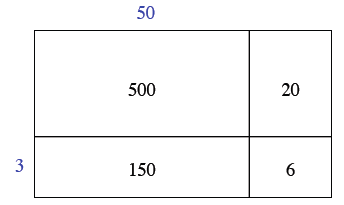### Home > CC1 > Chapter 2 > Lesson 2.3.4 > Problem2-86

2-86.

Look at the generic rectangle at right. Homework Help ✎

1. What two numbers are being multiplied using this rectangle? How can you tell?

We can see that 3 is a factor of both 150 and 6, so we can put it on the diagram as shown. Then we can see that 50 belongs at the top.2. Write the product as a sum of the areas and find the answer.

Remember that you are adding the smaller areas to find the total area.

3. Use the Distributive Property to write an equation showing that the product is equal to an expression with parentheses, multiplication, and addition.

• Refer to the Math Notes box in this lesson for help with the Distributive Property.

 500 20 150 6